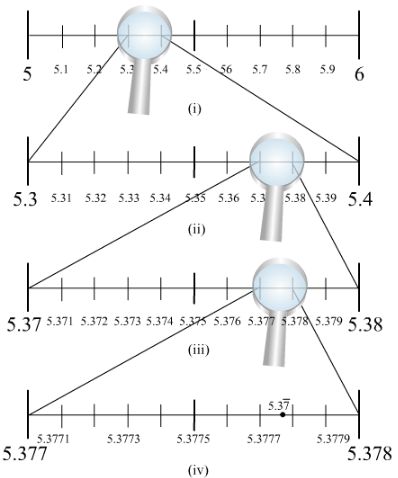# Visualise the representation of 5.37¯ on the number line upto 5 decimal places,

Question:

Visualise the representation of $5.3 \overline{7}$ on the number line upto 5 decimal places, that is upto $5.37777$.

Solution:We know that $5.3 \overline{7}$ will lie between 5 and 6 . So, we locate $5.3 \overline{7}$ between 5 and 6 . We divide this portion of the number line between 5 and 6 into 10 equal parts and use a magnifying glass to visualize $5.3 \overline{7}$.

$5.3 \overline{7}$ lies between $5.37$ and $5.38$. To visualize $5.3 \overline{7}$ more accurately we use a magnifying glass to visualize between $5.377$ and $5.378$.

Again, we divide the portion between $5.377$ and $5.378$ into 10 equal parts and visualize more closely to represent

$5.3 \overline{7}$ as given in the figure. This is located between $5.3778$ and $5.3777$.